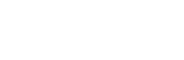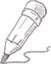#CDA持证人阿涛哥

2023-11-01   阅读量: 212

Mysql

## CDA一级SQL学习之要查询“计算机” 系的没有不及格成绩的学生学号和姓名A.SELECT Sno, Sname FROM S WHERE Sdept='计算机' INTERSECT SELECT Sno, Sname FROM SC WHERE GRADE>=60;

B.SELECT Sno, Sname FROM S WHERE Sdept='计算机' and Sno not in (SELECT SNO FROM SC WHERE GRADE<60);

C.SELECT Sno, Sname FROM S WHERE Sdept='计算机' EXCEPT SELECT SNOFROM SC WHERE GRADE<60;

D.SELECT Sno, Sname FROM S WHERE Sdept='计算机' and SNO in (SELECT SNO FROM SC WHERE GRADE>=60);A. SELECT Sno, Sname FROM S WHERE Sdept='计算机’INTERSECT SELECT Sno, Sname FROM SC WHERE GRADE>=60;

B. SELECT Sno, Sname FROM S WHERE Sdept='计算机' and Sno not in (SELECT SNO FROM SC WHERE GRADE<60);

C. SELECT Sno, Sname FROM S WHERE Sdept='计算机’ EXCEPT SELECT Sno FROM SC WHERE GRADE<60;

D. SELECT Sno, Sname FROM S WHERE Sdept='计算机’ and SNO in (SELECT SNO FROM SC WHERE GRADE>=60);

##建表建数实践：

#创建S表：

#DROP TABLE S;

CREATE TABLE S (

Sno CHAR(10) PRIMARY KEY,

Sname VARCHAR(20) NOT NULL,

Sdept VARCHAR(20) NOT NULL,

Sage INT NOT NULL

);

INSERT INTO S VALUES ('S001', '张三', '计算机', 20);

INSERT INTO S VALUES ('S002', '李四', '数学', 21);

INSERT INTO S VALUES ('S003', '王五', '计算机', 19);

INSERT INTO S VALUES ('S004', '赵六', '物理', 20);

INSERT INTO S VALUES ('S005', '钱七', '计算机', 22);

#创建C表：

#DROP TABLE C;

CREATE TABLE C (

Cno CHAR(10) PRIMARY KEY,

Cname VARCHAR(20) NOT NULL

);

INSERT INTO C VALUES ('C001', '数据库');

INSERT INTO C VALUES ('C002', '数据结构');

INSERT INTO C VALUES ('C003', '计算机组成原理');

INSERT INTO C VALUES ('C004', '操作系统');

INSERT INTO C VALUES ('C005', '计算机网络');

#创建SC表：

#DROP TABLE SC;

CREATE TABLE SC (

Sno CHAR(10) NOT NULL,

Cno CHAR(10) NOT NULL,

PRIMARY KEY (Sno, Cno),

FOREIGN KEY (Sno) REFERENCES S(Sno),

FOREIGN KEY (Cno) REFERENCES C(Cno)

);

INSERT INTO SC VALUES ('S001', 'C001', 66);

INSERT INTO SC VALUES ('S001', 'C002', 55);

INSERT INTO SC VALUES ('S001', 'C003', 44);

INSERT INTO SC VALUES ('S002', 'C001', 75);

INSERT INTO SC VALUES ('S002', 'C002', 80);

INSERT INTO SC VALUES ('S002', 'C003', 70);

INSERT INTO SC VALUES ('S003', 'C001', 90);

INSERT INTO SC VALUES ('S003', 'C002', 95);

INSERT INTO SC VALUES ('S003', 'C003', 85);

INSERT INTO SC VALUES ('S004', 'C001', 70);

INSERT INTO SC VALUES ('S004', 'C002', 80);

INSERT INTO SC VALUES ('S004', 'C003', 75);

INSERT INTO SC VALUES ('S005', 'C001', 85);

INSERT INTO SC VALUES ('S005', 'C002', 90);

INSERT INTO SC VALUES ('S005', 'C003', 80);

A.SELECT Sno, Sname FROM S WHERE Sdept='计算机' INTERSECT SELECT Sno, Sname FROM SC WHERE GRADE>=60;

B.SELECT Sno, Sname FROM S WHERE Sdept='计算机' and Sno not in (SELECT SNO FROM SC WHERE GRADE<60);

C.SELECT Sno, Sname FROM S WHERE Sdept='计算机' EXCEPT SELECT SNOFROM SC WHERE GRADE<60;

D.SELECT Sno, Sname FROM S WHERE Sdept='计算机' and SNO in (SELECT SNO FROM SC WHERE GRADE>=60);

0.0000 0 0 关注作者 收藏

## 评论(0)